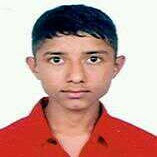# Numerical Method (SH553) - Question Paper 2073 Bhadra B.Agri II/II

 Subject:- Numerical Method (SH553)
 Exam Regular Level BE Full Marks 80 Program B. Agri Pass mark 32 Year/part II/II Time 3 hrs

 Tribhuwan University Institute of Engineering Examination Control Division 2073 Bhadra

ü  Candidates are required to give their answer in their own words as far as practicable.

ü  Attempt All questions.

ü  The figures in the margin indicate Full marks.

ü  Assume suitable data if necessary.

1.     Discuss the difference between absolute error and relative error with appropriate examples. 

2.     Write an algorithm of Secant method for finding a real root of a non linear equation. 

3.     Find a real root of the equation sinx=correct up to four decimal places using N-R method. What are the limitations of this method?

4.     Apply Gauss Seidal Iterative Method to solve the linear equations correct to 2 decimal places. 

10x+y-z=11.19, x+10y+z=28.08, -x+y+10z=35.61

5.     Find the dominant Eigen value and the corresponding Eigen vector of the given matrix using power method.6.     What is the practical significance of the least square method of curve fitting? Describe the normal equations to fit a given set of data to a linear equation y=ax+b [2+6]

7.     Using stirling formula find, given;8.     Estimate the value of cos(1.74) from the following data: 

 x 1.7 1.74 1.78 1.82 1.86 Sin(x) 0.9916 0.9857 0.9781 0.9691 0.9585

9.     Evaluateusing the three point Gaussian quadrature formula. 

10.                                                                                                                                                                                                                       Solve the following simultaneous differential equation using Runge-Kutta second order method at x=0.1 and 0.2 dy/dx =xz+1; dz/dx=-xy with initial conditions y(0)=0, z(0)=1 

11.                                                                                                                                                                                                                       Write a program in any high level language (C/C++/FORTAN) to solve a first order initial value problem using classical RK-4 Method. 

12.                                                                                                                                                                                                                       Solve the elliptic equationon the square mesh bounded byThe boundary values are u(x,0)=10, u(x,3)=90,and u(0,y)=70, u(3,y)=0,  0<y<3

***